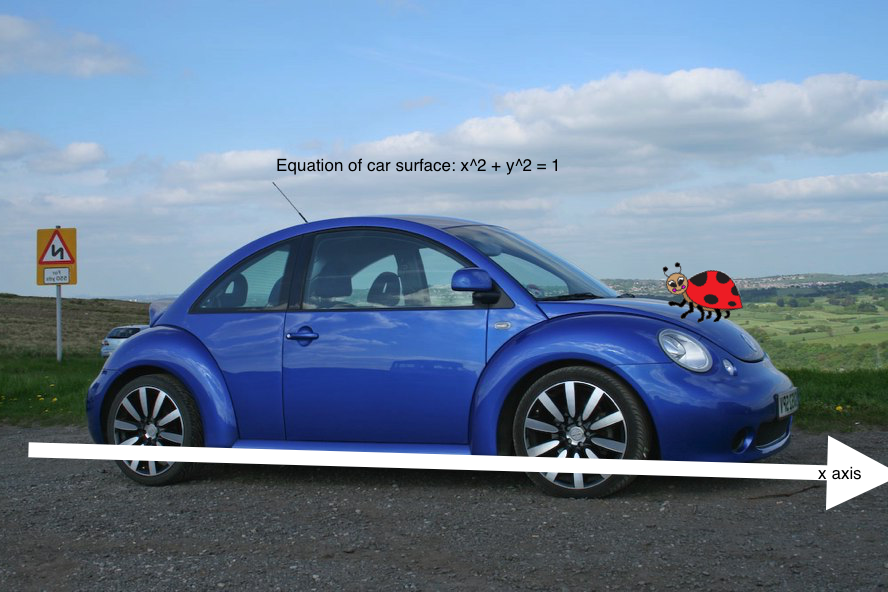I wish the car stayed still: part II

Calculus Level pendingA ladybug is climbing on a Volkswagen Beetle (a type of car). In its starting position, the surface of the car is represented by the unit semicircle $x^{2} + y^{2} = 1, y \geqslant 0$ in the $xy$-plane. The $x$-axis is the road. At time $t = 0$ the ladybug starts at the front bumper $(1, 0)$, and crawls counterclockwise on the surface of the car at a unit speed relative to the car. At the same time, the car moves to the right at speed 10.

What is the total distance traveled by the bug on the interval of time $0 \leqslant t \leqslant \dfrac{\pi}{4}$? If total distance is $\mathfrak{E}$, find $\left \lfloor 1000 \mathfrak{E} \right \rfloor$.

×